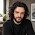# not only bridges

Engineering is not only bridges but a logical way of thinking

### Fast square roots

I have always been puzzled about the way square roots calculations are taught in the elementary school. I remember a very cumbersome method in which each decimal was extracted with hard work.

In college, especially in my studies in computer science, I have not always been permitted to use a calculator during the examination time, so I learnt to calculate square roots with pencil and paper in a sufficiently approximate but fast way. In my case, I use to apply the Newton-Raphson algorithm to the equation f(x) = x-root(K) = 0

Suppose we need to calculate the root of a real number K. To calculate the root of K, first assume an educated guess x.

For small numbers my usual estimate is x = K/2
Up to 1000, to start with x = 8+(K/40) usually works well. In other cases, bearing in mind some perfect squares helps.

Once the first estimate is made, it is refined with the formula
x[i+1] = (1/2) * (x[i] + (K / x[i]))

For example, if you are on-site and you do not have a calculator near you and a worker asks you about the side of a slab of 500 sq meters you know that the side L = root(500) = 10*root(5) = 10*x
then
x = 5/2 = 2.5 (error 12%)
x = 9/4 = 2.25 (error 1%)
x = 161/72 = 2.2361 ... (error 0.002%)

therefore the side is L = 22,361 m

The difference for root(780) between the classic algorithm and the Newton-Raphson one is clear in the appended images below.

Elementary school algorithm

Iterative algorithm

1.This is super useful and something that I was not ever taught in a math or engineering course at school... good to keep in the pocket though.

1.Thanks for your comment.

2.I thought that this method is called Babylonian method, used by Heron of Alexandria cca 1600yrs ago, which also seems to have been known by Babylonians 2000 yrs before Heron.
Am I wrong in my assertion?

1.Dear Eugen: you're absolutely right. Newton-Raphson for the square root is equivalent to the Babylonian rule. The good thing about Newton-Raphson is that you can get also cube roots, fourth roots, etc...

Also, the presented algorithm is equivalent to the following recursive function:

float sqroot (float num, float guess) {
if (num < 0.0) return -1.0;
if ((guess * guess) == num) return guess;
return sqroot (num, (guess + (guess / num)) / 2);}

3.super cool stuff. No one teaches these tricks at schools and colleges. Awesome work bro. Thank you for sharing :)